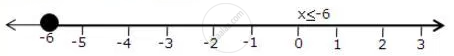Share

Represent the Solution of the Given Inequalities on the Real Number Line X + 3 <= 2x + 9 - ICSE Class 10 - Mathematics

ConceptLinear Inequations in One Unknown

Question

Represent the solution of the given inequalities on the real number line

x + 3 <= 2x + 9

Solution

x + 3 <= 2x + 9

-6 <= x

The solution on number line isIs there an error in this question or solution?

APPEARS IN

Solution Represent the Solution of the Given Inequalities on the Real Number Line X + 3 <= 2x + 9 Concept: Linear Inequations in One Unknown.
S